## Physics Formulae

Values are given to three significant figures, except where more are useful.

 speed of light in a vacuum c 3.00 × 108 m s–1 permittivity of free space ε0 8.85 × 10–12 C2 N–1 m–2 (F m–1) elementary charge e 1.60 × 10–19 C Planck constant h 6.63 × 10–34 J s gravitational constant G 6.67 × 10–11N m2 kg–2 Avogadro constant NA 6.02 × 1023 mol-1 molar gas constant R 8.31 J mol-1 K–1 Boltzmann constant k 1.38 × 10-23 J K–1 electron rest mass me 9.11 × 10-31 kg proton rest mass mp 1.673 × 10-27 kg neutron rest mass mn 1.675 × 10-27 kg alpha particle rest mass ma 1.646 × 10-27 kg acceleration of free fall g 9.81 m s–2

Formula

NameWave Equation

v= velocity  (m/s)
f = frequency (hz)
l = wavelength (m)Intensity Law

I = intensity (no units)
d = distance (m)Snell’s Law

v1 = speed in first medium
v2 = speed in second medium
sin i = angle in first medium
sin r = angle in second medium

• Angles are always measured to the normalElectric Field

E = Electric field (N/C, V/m)
F = Force (N)
q = charge (C)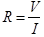Ohm’s Law

R = resistance (W)
V = voltage (V)
I = current (A)

Energy = VIt

Electrical Energy

Energy (J)
V = voltage (V)
I = current (A)
T = time (s)

P=VI

Electrical Power

P = power (W)
V = voltage (V)
I = current (A)Average Velocity

vav = average velocity (m/s)
r = distance covered (m)
t = time (s)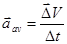Average Acceleration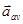= average acceleration (ms-2)
dv = change in velocity (m/s)
dt = change in time (s)Newton’s Second Law= sum of all forces (N)
m = mass (kg)= acceleration (ms-2)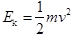Kinetic Energy

Ek = kinetic energy (J)
m= mass (kg)
v = speed (m/s)Momentum

P = momentum (Ns, kgm/s)
m = mass (kg)
v = velocity (m/s)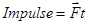Impulse

Impulse = change in momentum (Ns, kgm/s)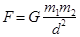Universal Gravitation

F = force (N)
G = universal gravitation constant (6.67 X 10-11 Nm2 kg-2)
m1 = mass of body 1 (kg)
m2 = mass of body 2 (kg)
d = separation between the two bodies (m)Kepler’s Third Law

r = radius of motion (m)
T = period of motion (s)
G = universal gravitation constant (6.67 X 10-11 Nm2 kg-2)
M = mass of system (kg)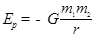Gravitational Potential Energy

Ep = Potential energy
G = universal gravitation constant (6.67 X 10-11 Nm2 kg-2)
m1 = mass of body 1 (kg)
m2 = mass of body 2 (kg)

r = separation between the two bodies from infinity to r (m)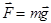Gravitational Force

F = force (N)
M = mass (kg)

g = gravitational constant at the surface of the Earth 9.81 ms-2Newtons’ Laws of Motion

ux = initial speed in x direction (m/s)
vx = final speed in x direction (m/s)
uy = initial speed in y direction (m/s)
vy = final speed in y direction (m/s)
a = constant acceleration (ms-2)
dx, dy = change in displacement (m)

t = time (s)Kepler’s third law

r = radius of motion (m)
T = period of motion (s)
G = universal gravitation constant (6.67 X 10-11 Nm2 kg-2)

M = mass of system (kg)Relativistic Length Contraction

Lv = apparent length (m)
Lo = “rest” length (m)
v = relative velocity (m/s)

c = speed of light (3 X 108 m/s)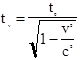Relativistic time dilation

tv = apparent time (s)
to = “rest” time (s)
v = relative velocity (m/s)

c = speed of light (3 X 108 m/s)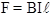Magnetic force on a current-carrying wire of length l in a magnetic field
F = force (N)
B= Magnetic Field (T)
I = current (A)
l = length (m)Force per unit length F = force (N)
l = length (m) per unit
I1, I2 = two currents
parallel=repulsive, antiparallel=attractive
d = separation of the two currents (m)
k=magnetic constant (2 X 10-7 NC-1m-1)torque t =torque (Nm)
F =force (N)
d=distance (m)torque on a coil immersed in a magnetic field

t =torque (Nm)
n =number of turns of coil
B=magnetic field (T)
I = current (A)
A = area of coil immersed in magnetic field (m2)

cos Q=angle between the coil and the magnetic field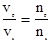Transformer equation

Vp = primary voltage (V)
Vs = secondary voltage (V)
Np = number of turns in the primary coil

Ns = number of turns in the secondary coil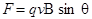Magnetic force on a charge in a magnetic field

F = force (N)
q = charge (C)
v = velocity (m/s)
B = magnetic field (T)

Sin Q = angle between the velocity and the magnetic field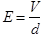Electric Field

E = Electric Field (N/C, V/m)
V = Voltage (V)

d = distance (m)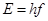Energy of a Photon

E = Energy (J)
h = Planck’s constant 6.626 X 10-34 Js

f = frequency (Hz)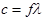Wave Equation

c = speed of light 3 X 108 m/s
f = frequency (Hz)

l = wavelength (m)